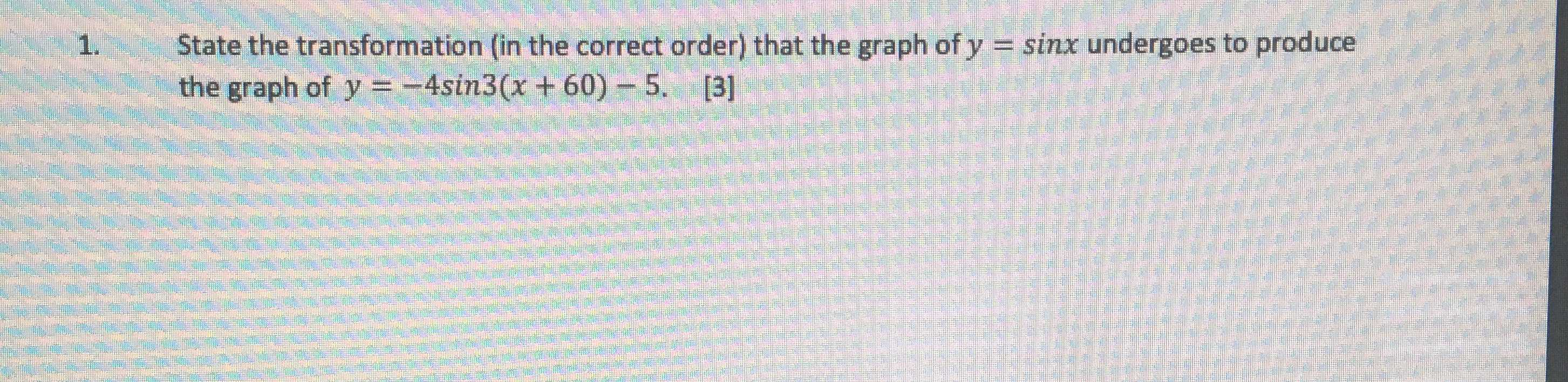### Still have math questions?

Algebra
Question1. State the transformation (in the correct order) that the graph of $$y = \sin x$$ undergoes to produce the graph of $$y = - 4 \sin 3 ( x + 60 ) - 5$$ .